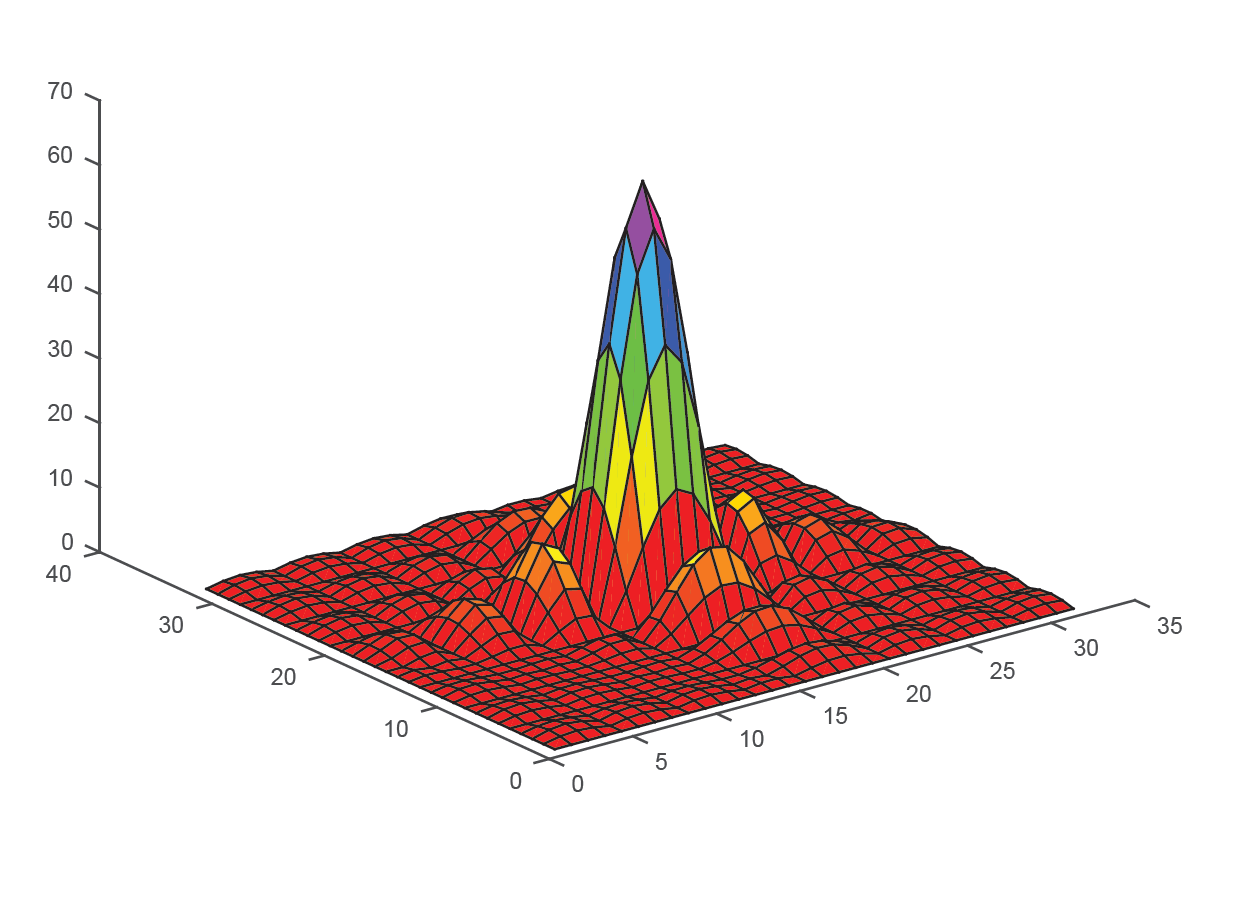MATLAB

What version of MATLAB is available on OSCER resources?

To find out which version of MATLAB is currently available on OSCER resources:

• Type module avail MATLAB
• You will get a result similar to this (with the version available at the time):Who can use MATLAB on OSCER resources?

The following users are allowed to access MATLAB on OSCER resources:

• Active OU faculty/staff/students with valid OU Norman campus accounts are allowed use the OU-licensed MATLAB.
• Visiting scholars/researchers/professors/affiliates/etc. also qualify to use MATLAB, as long as they have valid OU Norman campus accounts during their involvement with OU. However, they are restricted to using it for OU purposes only (basically, for the reasons why they are at OU).
• Retirees are not eligible to use MATLAB.
Please Note: OU-licensed MATLAB can only be used for educational/classroom purposes and for academic research in which OU is directly involved. It cannot be used for commercial purposes (even by eligible OU users), nor for academic and research purposes at other institutions.

• Type id
• If you find matlab under groups, you are already able to run MATLAB

Using MATLAB Interactively is FORBIDDEN

Running interactively on the login nodes could negatively impact performance for other users. You are FORBIDDEN to use MATLAB interactively. This means you are not allowed to type matlab at the Unix command prompt. Instead, ALL MATLAB runs MUST be performed via the batch scheduler. We will cancel jobs running interactively on the login nodes if we determine they are causing performance issues.

Sample Code

Step 1: Create a sample Matlab file called matlab_test.m and insert the following lines of code:

echo on;
colormap(hsv);
x = zeros(32);
x(13:20,13:20) = ones(8);
mesh(x);
y = fft2(x);
z = real(sqrt(y.^2));
w = fftshift(z);
surf(w);
grid;
echo off;
print -dpdf matlab_test_output.pdf;
quit;

Step 2: Create a batch submission file called matlab_batch.sh and insert the following lines of code:

#!/bin/bash
#
#SBATCH --partition=normal
#SBATCH --job-name=matlab_test
#SBATCH --output=matlab_output_%J.txt
#SBATCH --error=matlab_error_%J.txt
#SBATCH --time=12:00:00
#SBATCH --nodes=1
#SBATCH --mem=1024M